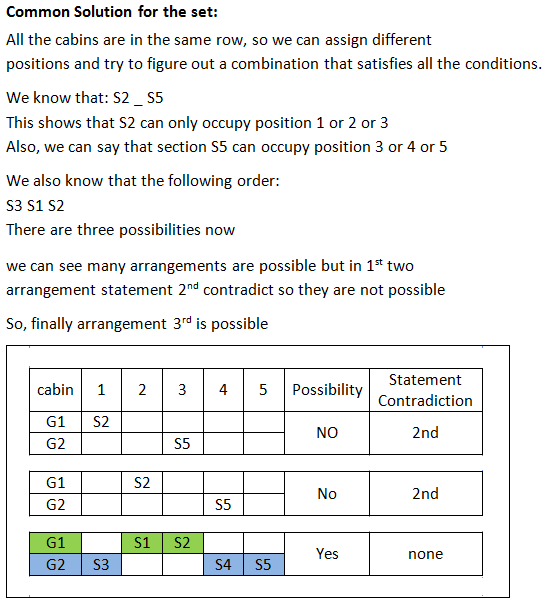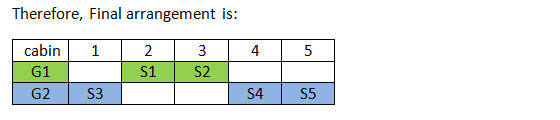### Logical Reasoning maths: Logical Reasoning maths type questions that you should solve before exams

Logical Reasoning maths type question set are usually asked from the following chapters:
• Permutation and combination
• Sequence and series
• Probability
• Geometry (Painted faces of a cube)
Logical Reasoning maths tests your analytical abilities (logic) and quantitative skills at the same time. Practice a variety of Logical Reasoning maths questions from the possible topics, as with little effort here, you can score really well.

Directions for the question set:
A company has five sections: S1, S2, S3, S4 and S5
Each section has been assigned a different cabin and all the cabins are in one row.
Cabins are numbered from 1 to 5.
1. There is only one cabin between S2 and S5 with S5 following S2.
2. S1 is placed just after S3 with S1 placed just before S2
3. The five sections are subdivided into two groups G1 and G2.
4. G1 consists of 2 sections S1 and S2, G2 contains 3 sections S3, S4 and S5.

Question 1: The fifth cabin belongs to which section?
(a) S1
(b) S2
(c) S3
(d) None of these

Question 2: Cabin number for Section S4 is:
(a) 5
(b) 4
(c) 3
(d) 2

Question 3: The cabins allotted to G2 group are:
(a) 1, 3 and 5
(b) 1, 4 and 5
(c) 2, 4 and 5
(d) 2, 3 and 4

### Answers and Explanations: Click the down arrow to expandAnswer 1: (d) Fifth cabin belongs to ‘S5’ section The correct option is (d).

Answer 2: (b) Section S4 belongs to cabin 4 The correct option is (b).

Answer 3: (b) We can see the form above order that Group G2, that is sections S3, S4 and S5 occupy cabin numbers 1, 4 and 5. The correct option is (b).

Extra tips for Logical Reasoning maths:
• As it is a Logical Reasoning maths combination question, the question may have a lot of data, read well and make a concise figure/table to simplify your solution.
• Make sure you account for the multiple cases/possibilities in your diagram. It is advisable to draw parallel diagrams and immediately rule out the case that fails.
• Be thorough with formulas, as not knowing any one of them that is required to crack the set, may lead to complete loss of a potential set.